# 记录一次执行计划恶化的处理过程

XLJ

（日期201639日）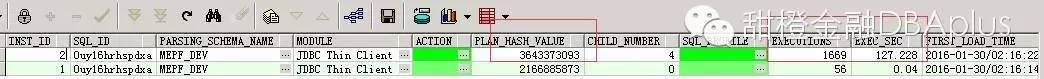0uy16hrhspdxa文本如下(语句中的表做了脱敏处理)

select *

from (select rownum no_, A_.*

from (select a.order_id as ACCEPTSEQNO,

to_char(a.acct_date, 'yyyymmdd') as ACCEPTDATE,

to_char(a.acct_date, 'hh24miss') as ACCEPTTIME,

(select to_char(decode(pay_money,

'0',

due_moeny,

pay_money) * 100)

from t_xxx_ordfee

where order_id = a.order_id) as TXNAMOUNT,

'XXX商户' as MERCHANTNAME,

(select (case

when l.prod_id = '111' then

decode(a.order_type,

'OT001',

'01',

'OT301',

'03',

'OT501',

'04')

when l.prod_id = '112' then

decode(a.order_type,

'OT001',

'02',

'OT301',

'05')

else

'null'

end)

from t_pdm_action l

where l.action_id = c.action_id) as TXNTYPE,

'08' as TXNCHANNEL,

(select value1

from t_xxx_act_attr t

where t.actlist_id = c.actlist_id

and t.attr_id = '1016') as GOODSNAME,

decode(:1,

'01',

'XXX商户消费',

'02',

'XXX商户充值',

'其它业务') as TXNDSCPT,

decode(a.stat,

'S0C',

'00',

'S0A',

'01',

'S0F',

'02',

'S0U',

'03',

'S0D',

'04',

'S0X',

'05',

'S0G',

'01',

'S0T',

'06',

'S0R',

'07',

'null') as ORDERSTAT,

decode(c.action_id,

'140',

'04',

'141',

'04',

'138',

'02',

'147',

'03',

'154',

'05',

'156',

'06',

'01') as ACCOUNT,

a.act_stat as BUSISTAT,

a.pay_stat as PAYSTAT

from t_xxx_order   a,

t_xxx_actlist c,

t_xxx_info    b,

t_xxx_partner d

where 1 = 1

and c.order_id = a.order_id

and a.cust_id = b.cust_id

and b.prtn_id = d.prtn_id

and (c.action_id in

(select action_id

from t_pdm_action

where prod_id = '111') or action_id = '0')

and a.acct_date >= trunc(to_date(:2, 'yyyymmdd'))

and a.acct_date < trunc(to_date(:3, 'yyyymmdd') + 1)

and c.obj_code = :4

and a.order_type != 'OT101'

and a.order_type != 'OT601'

and a.order_type != 'OT501'

and a.order_type != 'OT502'

order by a.acct_date desc) A_

where rownum < (to_number(:5) + to_number(:6) + 1)) B_

where B_.no_ > to_number(:7)

，下了地铁奔到单位，开机……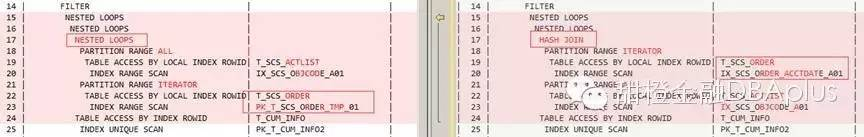sql本文中涉及t_xxx_order,t_xxx_actlist的语句：

from t_xxx_order  a,t_xxx_actlist c,

where c.order_id = a.order_id

and a.acct_date >= trunc(to_date(:2, 'yyyymmdd'))

and a.acct_date < trunc(to_date(:3, 'yyyymmdd') + 1)

and c.obj_code = :4

2.稳定执行计划

一般有几种办法

(1)绑定执行计划（sqlprofile SPM

(2)调整统计信息（收集新的统计信息、或者手工设定一个统计信息或者删除统计信息等）

(3)创建索引、不可见索引等涉及对象的操作

(4)改写优化sql语句（包括添加各类hint），但这无法立刻实施并上线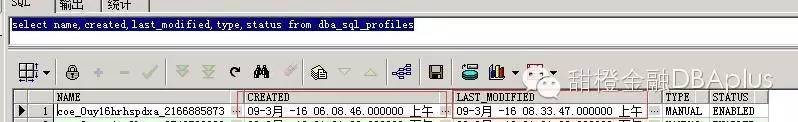exec dbms_stats.gather_index_stats(ownname=>'MEPF_DEV', indname=>'IX_XXX_ORDER_ACCTDATE_A01', estimate_percent=>10, degree=>4)

exec dbms_stats.gather_index_stats(ownname=>'MEPF_DEV', indname=>'PK_T_XXX_ORDER_TMP_01',estimate_percent=>10,degree => 8)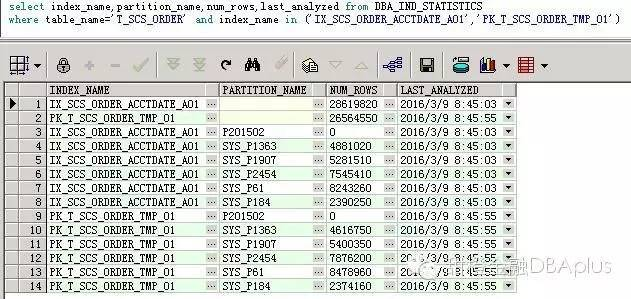Alter index IX_XXX_ORDER_ACCTDATE_A01 invisible;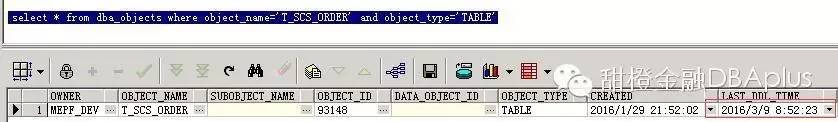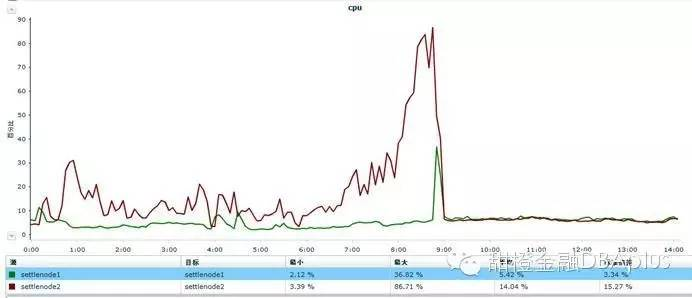CPU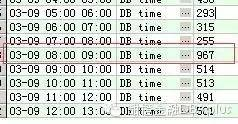DB time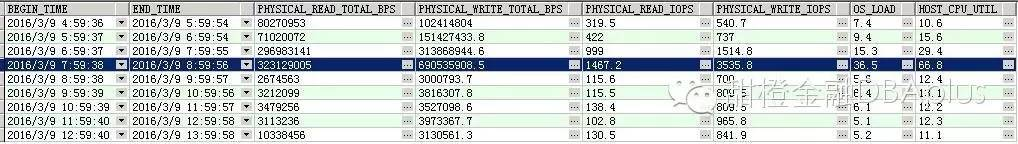IO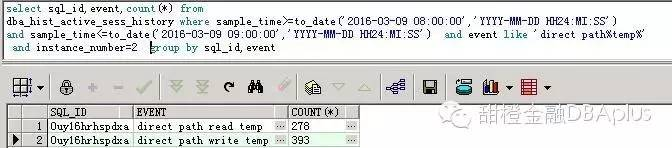wait event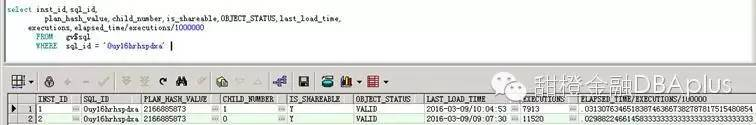1. sql确实有优化的空间（几张大表都可以添加分区条件列从而使得在分区范围内进行扫描），这个之前已反馈并且开发也已完成优化，正在安排上线时间

2.  类似的查询以后将剥离本系统，这种大sql马上会寿终正寝

1. 那么为什么会发生执行计划的变化呢？为什么就2节点？

2. 为什么sqlprofile无法绑定执行计划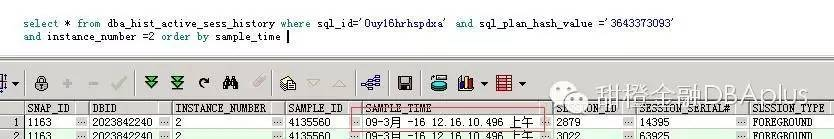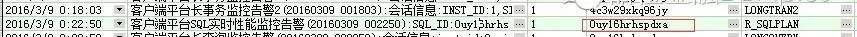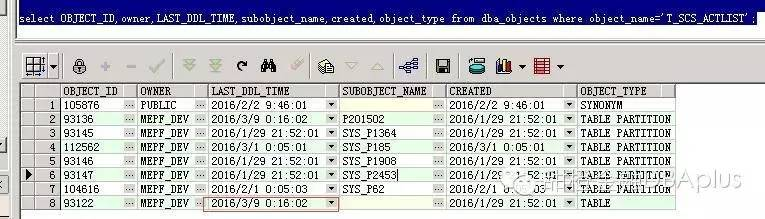t_xxx_aclistDDL造成sql重新解析，造成产生了新的低效的执行计划，访问了t_xxx_order表的其他索引

select column_name ,high_value,low_value,histogram from user_tab_columns where table_name='T_XXX_ORDER' and column_name in ('ACCT_DATE');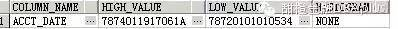select

a.column_name,

a.num_distinct,

display_raw(a.low_value,b.data_type) as low_val,

display_raw(a.high_value,b.data_type) as high_val,

b.data_type

from

dba_tab_col_statistics a, dba_tab_cols b

where

a.owner='MEPF_DEV' and

a.table_name='T_XXX_ORDER' and

a.table_name=b.table_name and

a.column_name=b.column_name and

a.column_name = 'ACCT_DATE'

and b.owner=a.owner

order by 1, 2;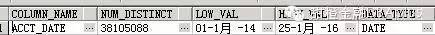1. 初次解析带入的绑定变量不同，我后来看了下，2个节点代入的绑定变量差别不大

2. 是因为当时2节点在批量数据归档和创建索引，负载非常高(这个就不举证了，凌晨DBA在做相关变更)，这可能会造成CBO没有足够的资源来判断和选择最优路径，dba_sql_profile看绑定正常

select s.instance_number,s.sql_id,s.plan_hash_value,s.sql_profile,t.begin_interval_time

from dba_hist_sqlstat s,dba_hist_snapshot t

where s.sql_id='0uy16hrhspdxa' and s.sql_profile is not null

and s.snap_id=t.snap_id

order by t.begin_interval_time; --没有记录  证明没有生效

select sql_profile from gv\$sql where sql_id='0uy16hrhspdxa'  --显示为空 证明没有生效

more coe_xfr_sql_profile_0uy16hrhspdxa_2166885873.sql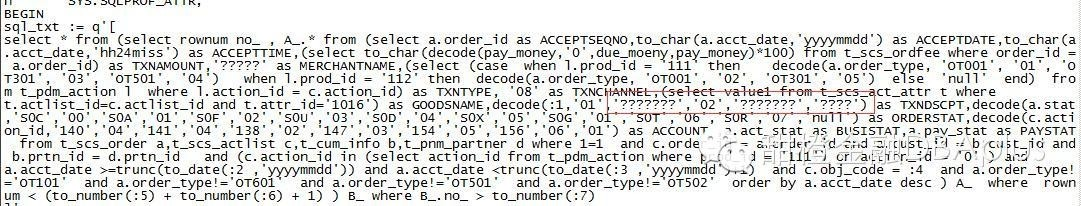• 博文量
126
• 访问量
991705